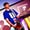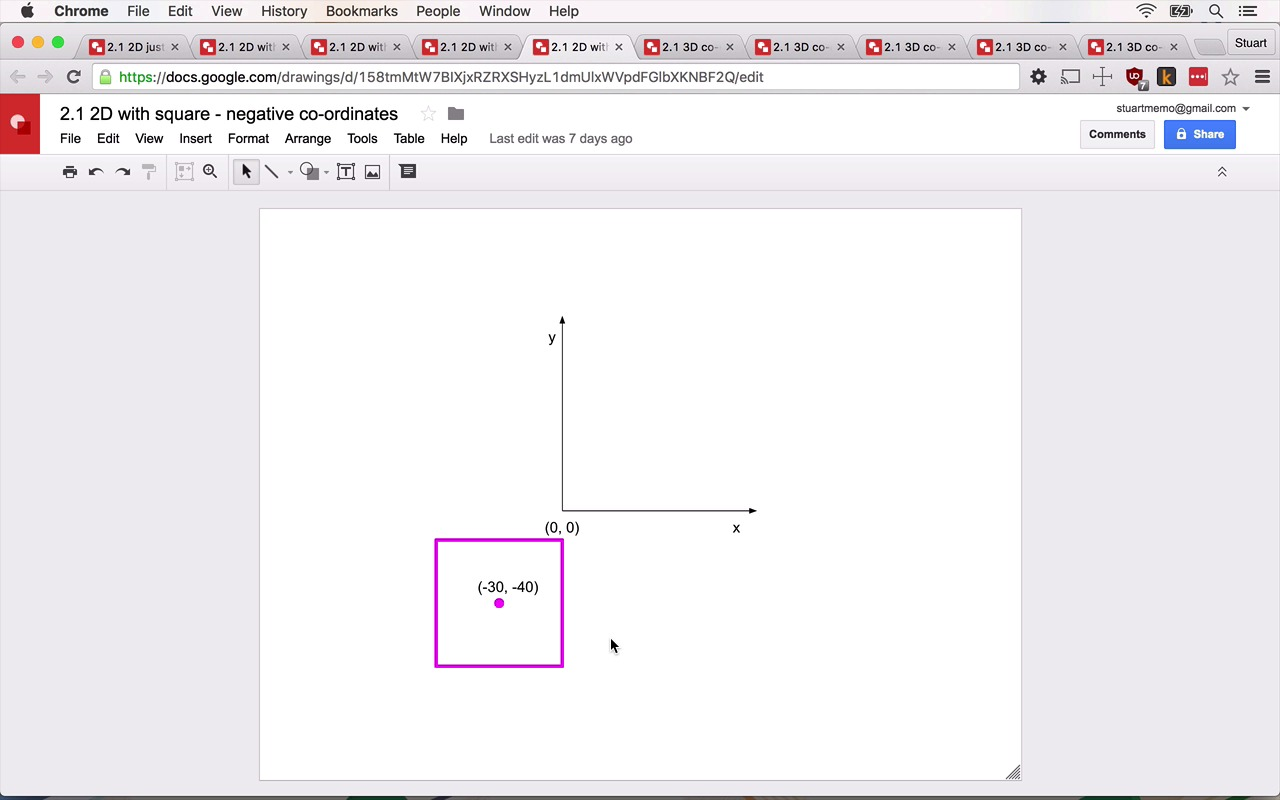Lessons: 10Length: 50 minutes• Overview
• Transcript

# 2.1 3D Concepts

In this lesson, you’ll learn some of the most important concepts for dealing with 3D—in or out of the browser!

## 4.Summary1 lesson, 01:07

### 2.1 3D Concepts

Okay, just hang on a moment. Before get going, let's go over some important three.js concepts you'll need to know before you're let loose on your digital canvas. The way we make things appear on screen the three.js is analogous to have a film director will shoot movie. First we need a scene, your scene is where everything takes place. If you want something to appear on screen, you're going to have to add it to your scene and when you add it to your scene, you're going to have to tell three where you want it to be using coordinates. Here we have our scene, we have an x-axis and y-axis. You're probably quite familiar with this concept. But I just want to highlight something. With three the, coordinates start in the middle of your screen, not in the top left as is usually the case with browsers. So if I wanted to draw a square in the middle of the scene, I would draw it at (0, 0). Also note, it's the center of the square that is a (0, 0), not the edge but the center. If I were to move the square up and right, the coordinates might be something like (60, 40). That's 60 pixels across and 40 pixels up. However, if we go the other way, our y-axis becomes negative. It will still be 60 pixels along but it would be -40. Similarly, if we moved our square to the negative x-axis, this would be (-30, -40). Okay, so we have an x and y-axis. But the whole point of three is that we can draw 3D graphics. So for that, we need a z-axis. Imagine drawing a line from your eyes right through your computer screen. That would be the z-axis. This is quite hard to visualize in 2D drawing, but if we were to draw a box at (0, 0, 0) because we've got to be coordinates now, it would still be about here. The middle of the box would be right where these axes cross. Now if we wanted the box to appear closer, then we would increase the value of the z coordinate. So here would be (0, 0, 10), for example. And if we wanted farther away, it would be (0, 0, -10). All these coordinates work together allowing us to place objects anywhere in a 3D space. So what else does a film director need? well, a camera would be quite useful, I imagine. So we'll need one of them. A camera in this instance, is used to capture our scene. Wherever we point the camera at, is what we'll see on screen. Like our box, we can place the camera anywhere in our scene facing any direction. Cameras aren't great without light, though, and currently we're completely in the dark. Luckily three provides us with a variety of different lights, that again we can move around the scene, pointing at things we want to shine light upon and therefore be revealed to the camera. Okay, that's enough theory, let's make something.

Back to the top